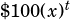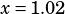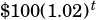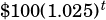Can you work through question 38 on Section 4 of practice test 1? Thanks 🙂

Sure thing! First, remember the info we had to use to solve #37. Jessica has an account that earns 2% interest compounded annually, and she makes one deposit of \$100. She uses the expressionto calculate the value of the account afteryears. In question #37, we had to figure out that, which represents that 2% growth.

As an aside, remember, when we set up an exponential expression for compound interest, we have to use. Below is the full setup, although we almost never have to worry aboutin SAT questions because interest will usually compound only once per period, as it does in this question.

OK, so now we’re ready for #38, in which we learn that Tyshaun has an account that’s exactly the same as Jessica’s, except it earns a little bit more interest: 2.5% instead of 2%. Remembering that Jessica’s account will be worthafteryears, we can say Tyshaun’s account will be worthafteryears.

So all that’s left for us to do is compare how much each account will be worth after 10 years. (Remember in your calculations to round to the nearest hundredth, since we’re talking dollars and cents here.)

Jessica:Tyshaun:Subtract to get the difference: